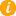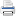# Math K-Plus

Math TestMath QuizQuiz Results• Option 2. Solve a calculator generated math problem. First press the NEW PROBLEM button. Next enter your answer, in to the calculator. Lastly, press the ADD button and then compare your answer with the calculator's.

Field Name Multiply Values
Multiplicand: You can enter only 5 digits
Multiplier: You can enter only 5 digits
×## Make the smaller number the multiplier and the larger number the multiplicand.

PROBLEM SOLVING STEP 0.

+84808
× +1381

84808
×  1381

PROBLEM SOLVING STEP 1.

84808
×  1381
8

PROBLEM SOLVING STEP 2.

84808
×  1381
08

PROBLEM SOLVING STEP 3.

84808
×  1381
808

PROBLEM SOLVING STEP 4.

84808
×  1381
4808

PROBLEM SOLVING STEP 5.

84808
×  1381
84808

PROBLEM SOLVING STEP 6.

84808
×  1381
84808

PROBLEM SOLVING STEP 7.

6
84808
×  1381
84808
4

PROBLEM SOLVING STEP 8.

6
84808
×  1381
84808
64

PROBLEM SOLVING STEP 9.

6 6
84808
×  1381
84808
464

PROBLEM SOLVING STEP 10.

36 6
84808
×  1381
84808
8464

PROBLEM SOLVING STEP 11.

636 6
84808
×  1381
84808
78464

PROBLEM SOLVING STEP 12.

84808
×  1381
84808
678464

PROBLEM SOLVING STEP 13.

2
84808
×  1381
84808
678464
4

PROBLEM SOLVING STEP 14.

2
84808
×  1381
84808
678464
24

PROBLEM SOLVING STEP 15.

2 2
84808
×  1381
84808
678464
424

PROBLEM SOLVING STEP 16.

12 2
84808
×  1381
84808
678464
4424

PROBLEM SOLVING STEP 17.

212 2
84808
×  1381
84808
678464
54424

PROBLEM SOLVING STEP 18.

84808
×  1381
84808
678464
254424

PROBLEM SOLVING STEP 19.

84808
×  1381
84808
678464
254424
8

PROBLEM SOLVING STEP 20.

84808
×  1381
84808
678464
254424
08

PROBLEM SOLVING STEP 21.

84808
×  1381
84808
678464
254424
808

PROBLEM SOLVING STEP 22.

84808
×  1381
84808
678464
254424
4808

PROBLEM SOLVING STEP 23.

84808
×  1381
84808
678464
254424
84808

PROBLEM SOLVING STEP 24.

84808
×  1381
84808
678464
254424
84808
+117119848